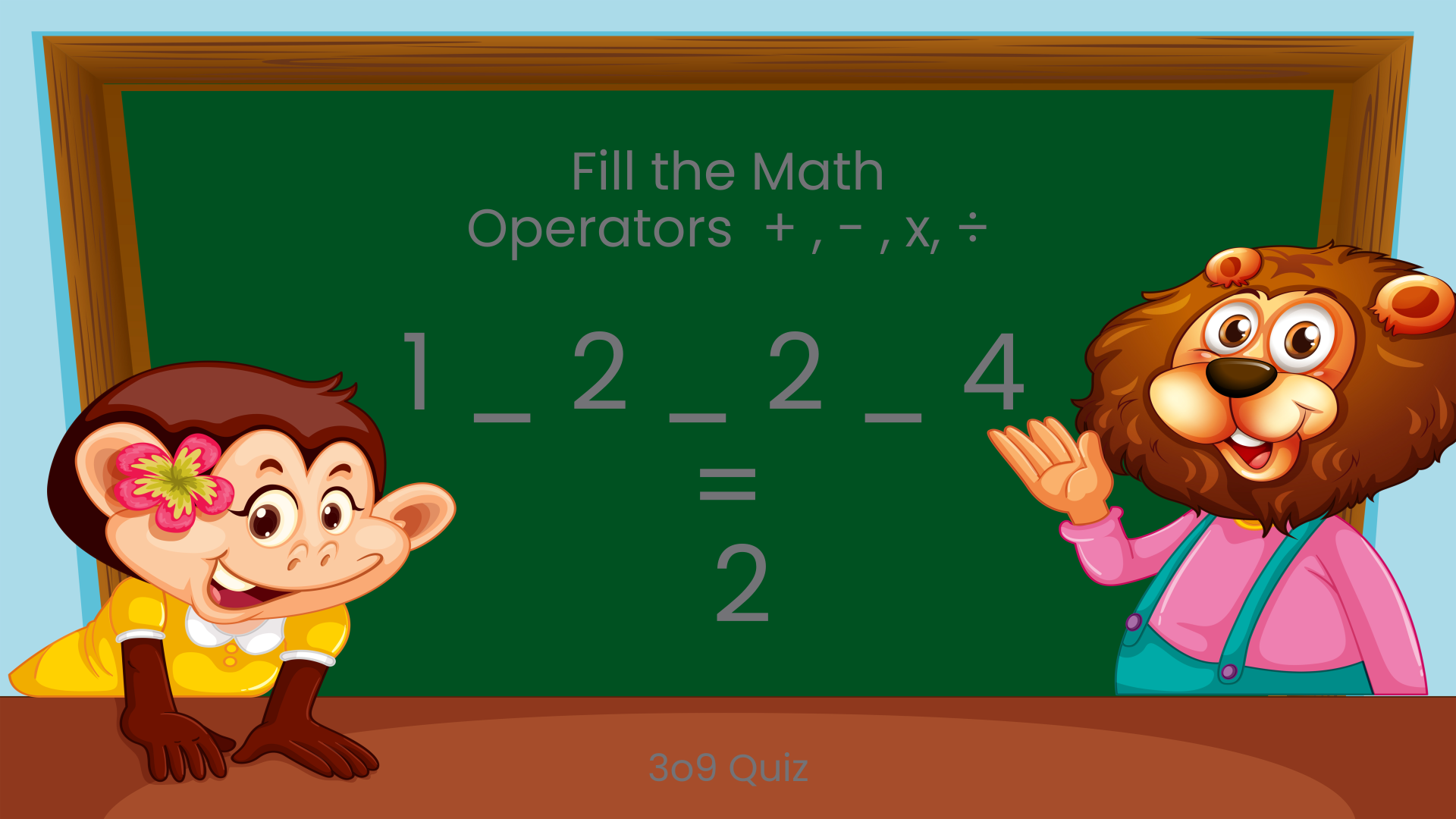# Can You Complete This Mathematical Equation? : 1 _ 2 _ 2 _ 4 = 2

To solve this puzzle, you need to have quick-wittedness and problem solving capacity. An equation with three blank operators will be given and you'll need to identify the missing operators and complete the equation. This is a great way to evaluate your grasp of basic mathematics and refine your logical skills. Get ready to put your brain to work and enjoy the challenge!Can You Complete This Mathematical Equation? : 1 _ 2 _ 2 _ 4 = 2